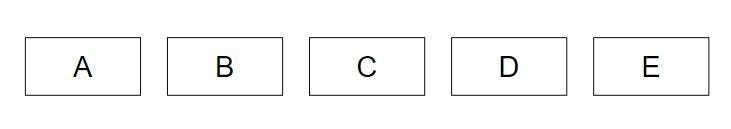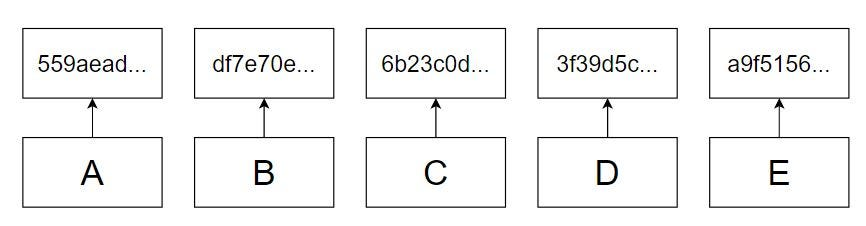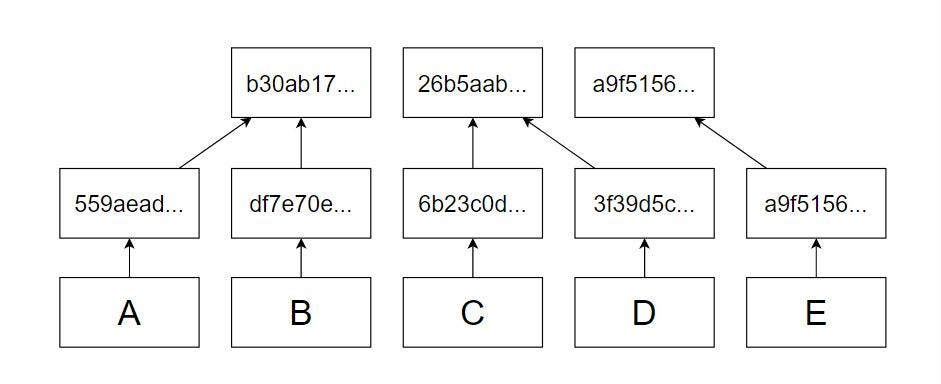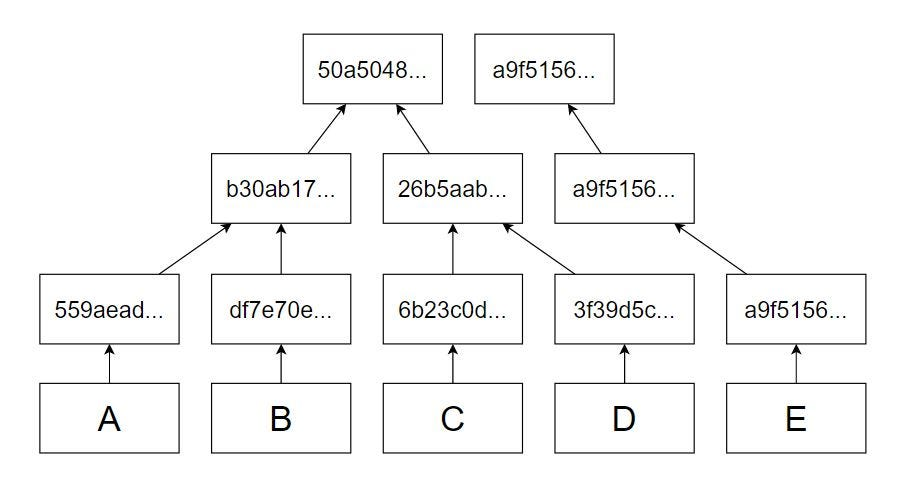# Implementing Merkle Tree and Patricia Trie

0
68### Introduction

Merkle and Patricia are the two most popular data structures used in the Ethereum Blockchain with Bloom Filters very close behind it. In this article I will cover the following:

1. About Merkel Trees and Patricia Tries
2. Algorithm
3. Implementation in Node.js

### Merkel Trees

Merkel Tree isn’t a new concept in Computer Science, it has been around for decades and originates from the field of Cryptography.

To simply put, Merkel Trees are essentially a tree data structure in which data is stored in the leaf nodes and non leaf nodes store hashes of data with each non-leaf node being the combined hash value of the two nodes below it.

Mathematically, it can be expressed as

For example: Given a list of alphabets, create a merkel tree from it.

The bottom most layer of the tree would contain all the letters as the leaf nodes.Lowest layer of the tree would contain the data in each node

The layer above contains its hash values.The layer above the leaf node has the hash values of leaf node data

The nodes in the layer after the second layer are contains the hash value of the child nodes. Generally we take two nodes from the second layer and combine them to form another node. We can take more than two nodes as well but binary merkel trees is the simplest of them all and increasing the degree of nodes only increase the computation and algorithms complexity.

If we have even number of nodes, we take 2 consecutive nodes and form the parent layer. But if we have odd number of nodes, we take two consecutive nodes until one is left to form the parent layer, and then we repeat the remaining node by copying the hash to the parent layer.Layer 3 has the hash of the values of the 2 consecutive nodes of layer 2 and in case we have odd nodes in a layer the last node is repeated

Similarly the fourth layer is formed using the values of the third layer.The fourth layer is formed by the hash of the values of the 2 consecutive nodes of layer 2

The final layer or the root of the Merkel Tree is formed by hash value of the last two nodes remaining in top most layer. In any case, odd or even leaf nodes, we will always have two nodes in the top most layer.

#### Verification

The importance Merkel Trees is in its ability to verify data with efficiency. Given any data from the list we can verify in O(h) time complexity that this data is valid or not. Moreover, we do not need the entire list for verification.

A much simpler form of Merkel Tree is a hash chain or simply a blockchain in which each node has the hash of the previous node’s value. If we tamper any node in between we can identify in O(n) time whether the node is tampered or not. Verification in hash chain can be performed by calculating the hash of all the nodes starting from the node in question and go till the end. In a situation where we have with multiple nodes to verify, we start with the node that is first among all the suspected nodes and calculate the hash of the last node from then on. Now that we have the hash of last node, we can compare and check if this hash matches or not. Hash chain seems simple but is not an efficient choice for large data objects. Since we need the entire chain physically present with us to verify the data, it makes hash chains space inefficient as well.

This is not the case with verification in Merkel Tree. To illustrate the verification process consider the example below.

Suppose I received a data C from another server. Lets say this is C’. We want to verify C’ is not tampered. We have in out possession a merkel tree of all the data in the list.

In case of a hash chain we would need the entire list of data to verify that C’ is correct. In Merkel Tree we only need the hashes. Following diagram illustrates how we can verify, C’ without other data objects available with us.

1. Find the position of the C’ in the list. Probably by searching by id.
2. Calculate the the hash of C’
3. Calculate the value of the parent node by hashing the current node with its neighbor ( next if position is odd and previous if position in even) and set the parent as the current node.
4. Repeat step 3 until we find the root
5. Compare the root with the previous root, if they match then C’

Compare the new root with the existing root. If the new root matches then the C’ is essentially C and not tampered.

To verify a data in hash chain we need O(n) time since we would calculate n hashes in the worst case where as in case of Merkel Tree the same data can be verified in O(logn) time since we only calculate logn hashes.

#### Algorithms

This section describes the algorithms in mathematical form for creation and verification in Merkel Trees.

Creation

As already mentioned before, Merkel Tree are created by taking two nodes from each layer and hashing them to create the parent node. by representing the tree in matrix form we can mathematically write it as:

This makes the root of the tree available at tree

Verification

Verification is a bottom-up approach where we start from the data, find its hash and calculate the parent and continue this until we find the root. Mathematically, we can express it as follows:

#### Implementation

We will be implementing a Merkel Tree in Node.js

Prerequisites

1. Node.js
2. VS Code
3. Coffee

Code

Create your project directory and cd into it.

`mkdir merkel-and-patricia && cd merkel-and-patricia`

Open VS Code in this directory

`code .`

Before we implement our we need to create a function for hashing data. So create a file named helper.js and the following code in it.

We will be using this file for hashing our data in the rest to the project. Next we’ll create our Transaction class.

Transaction Class will contain the following properties:

1. to
2. from
3. amount
4. id
5. hash

Create a file Transaction.js and add the following code in it.

TODO: Transaction Class

The function getCountand incrementCountare used to provide the transaction an id. You may use uuids instead of this.

Inorder to store all the transaction we will create a transaction list class which will contain a array of transactions.

Create a file TransactionList.js and add the following code in it.

We have our hashing function and our data. Lets implement the Merkel Tree.

Create a file named MerkelTree.js and create a MerkelTree Class with only on property root which is the Matrix that will hold our entire tree.

In this class create a method with name createTree which takes only on parameter, TransactionList instance and creates a Merkel Tree from it.

createTree method will first take the transactionList add it to the bottom most layer and the transaction hashes right above them. Next it will take two items from the top most layer and hash them together and save in the temporary list until all the items are covered, in case a single item remains, it is pushed into the temporary array directly and the temporary list temp is added to the beginning of the root This process is repeated until the first item of the root has length equal to one, which would indicate that we have found the root hash.

Now that we have a tree created. Lets write a method to verify a transaction.

The verification will use the same algorithm described above, take the neighboring node and node to verify, hash them and move to the parent layer and do the same but the node to verify will the hash we calculated before.

Create a function verfiy in the merkel tree class, taking a single parameter, a transaction.

Our MerkleTree class is complete. Below is the entire code for the class.

To test the functionality create a js file in the root directory , name it test.js and add the following code in it.

It should print the following output:

`Element found at: 2ValidElement found at: 2Not Valid`

You can uncomment the console logs to print the entire root and tampered transaction.

This completes the section on Merkel Tree. Up next is Patricia Trie.

### Patricia Tries

Patricia Tries are n-ary trees which unlike Merkel Trees,is used for storage of data instead of verification.

To simply put, Patricia Tries is a tree data structure in which all the data is store in the leaf nodes, where each non-leaf nodes is a character of a unique string identifying the data. Using the unique string we navigate through the character nodes and finally reach the data.

Patricia Trie is like a hash table but with a few subtle differences.

Lets take a look at an example. Consider the following words:

Cat, Cats, Car, Dog, Dogs, Doggo, Ant

A patricia trie storing these items will look like this:

The node with value END denotes that the path traversed till now is actually a word. Those nodes that do not have END child node denotes that the word is not present.

For example, in the diagram above, the word ANT is present in the trie because of the END node after “T”. Similarly, for CATS the END node is present after S which makes it a word in the trie. Interestingly, if we put an END node before like for CAT, we’ll have two words store in the same path but we can access the CAT by not traversing all the way to the bottom and checking if END exists anywhere between.

So is the case with DOG,DOGS and DOGGO. For DOG we will have only one returned value since it has it has the END node. But if we search the patricia with DOG as a prefix we will get three returned values. That is basically, depth first search used here.

Ethereum uses Patricia tries for storing transactions in blocks, transaction receipts and maintaining state of the network.

#### Storage

For storing data object we don’t need prefixes like in case of words. Since our data objects are either transactions or blocks and all the “unique strings” that we would use to store our data in the trie would be the transaction hashes or block hashes, would always be of the same length, so we need not worry about prefixes.

If we create a Patricia trie for transactions it should look like the following, although a lot bigger:

Each transaction hash will have character either number or character, (depends on the algorithm). For sha256 we will 32 character long hash. If we assume that the hash is only made from 0–9 and A — Z, then each node in the patricia trie will have 35 child nodes. Starting from the root, we will traverse down while matching each character to the node value until the end where we will get our transaction data object.

#### Algorithm

Each node in the trie, we can say, is itself a hash table which hash a character as the key and another hash table as the value. All operations, insertion, deletion and access take O(h) where h is the length of hash or depth of the tree in our case.

By using this definition, we can write the following algorithm for storing data in the trie:

In this algorithm we create an empty key-value pair object, traverse the entire length of the hash and for each character set the value as a new empyt key-value pair object. Also for each charater we set the curr mapping to the next mapping. And finally when we have created the entire branch, we set the data at the end with the last node key a label “DATA”.

While accessing we return the value of the last mapping for the key “DATA” and in deletion we just delete the leaf node for the given hash.

#### Implementation

This section provides implement the Patricia Trie algorithm described above in Node.js. Create a file PatriciTrie.js and add the following code in it.

The methods add, get and remove do as they are named, insert transaction to the trie, access transaction from the trie and delete transaction from the trie respectively.

Let test this functionality. Add the following into the test.js file we created before.

Running this file you should be getting an output similar to the below output:

`\$ node testTransaction {  to: 0.01106239432861833,  from: 0.774577364867872,  amount: 0.7140173399739937,  id: 0,  hash:   'e4bc0c48be1ad748af6dbc714ddf49d3b76643a491d068a5f1494c84b54971ad' }     truenullnullfalse`

This completes the section on Patricia Tries.

### Extension

You may extend this project further by implementing the following:

1. Implement blockchain with Merkel Tree and Patricia Tries.
2. Implement state using Patricia Trie.
3. Test the working of the two data structures in areas other than blockchain.

In this article, we implemented a basic Merkel Tree and Patricia Trie used in Ethereum. The code for the article can be found in my github repository .